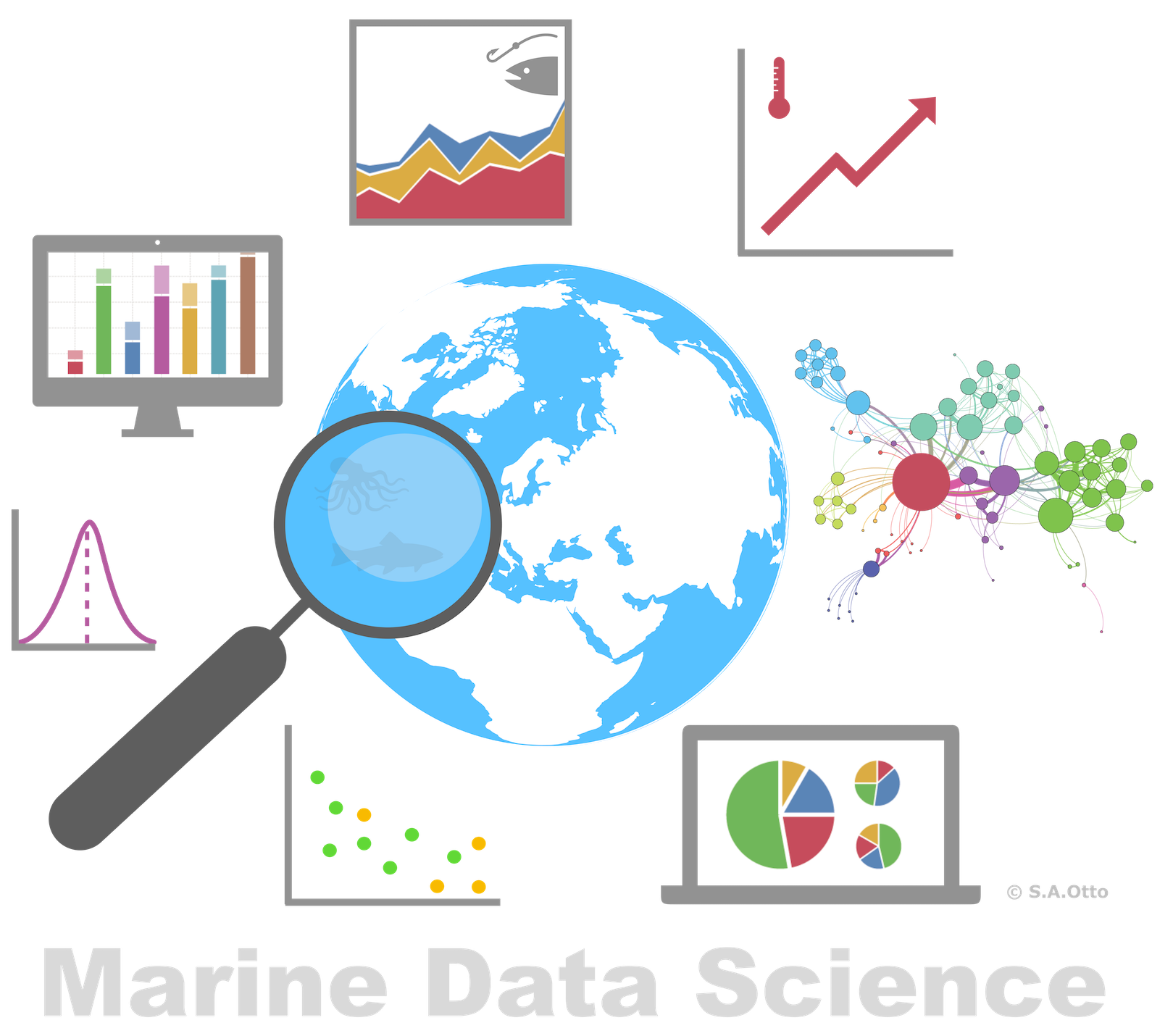Data Analysis with R

8 - Intro2Visualization - Part 1

Postdoctoral Researcher

Recap ...

Visualization is one of the corner stones of data science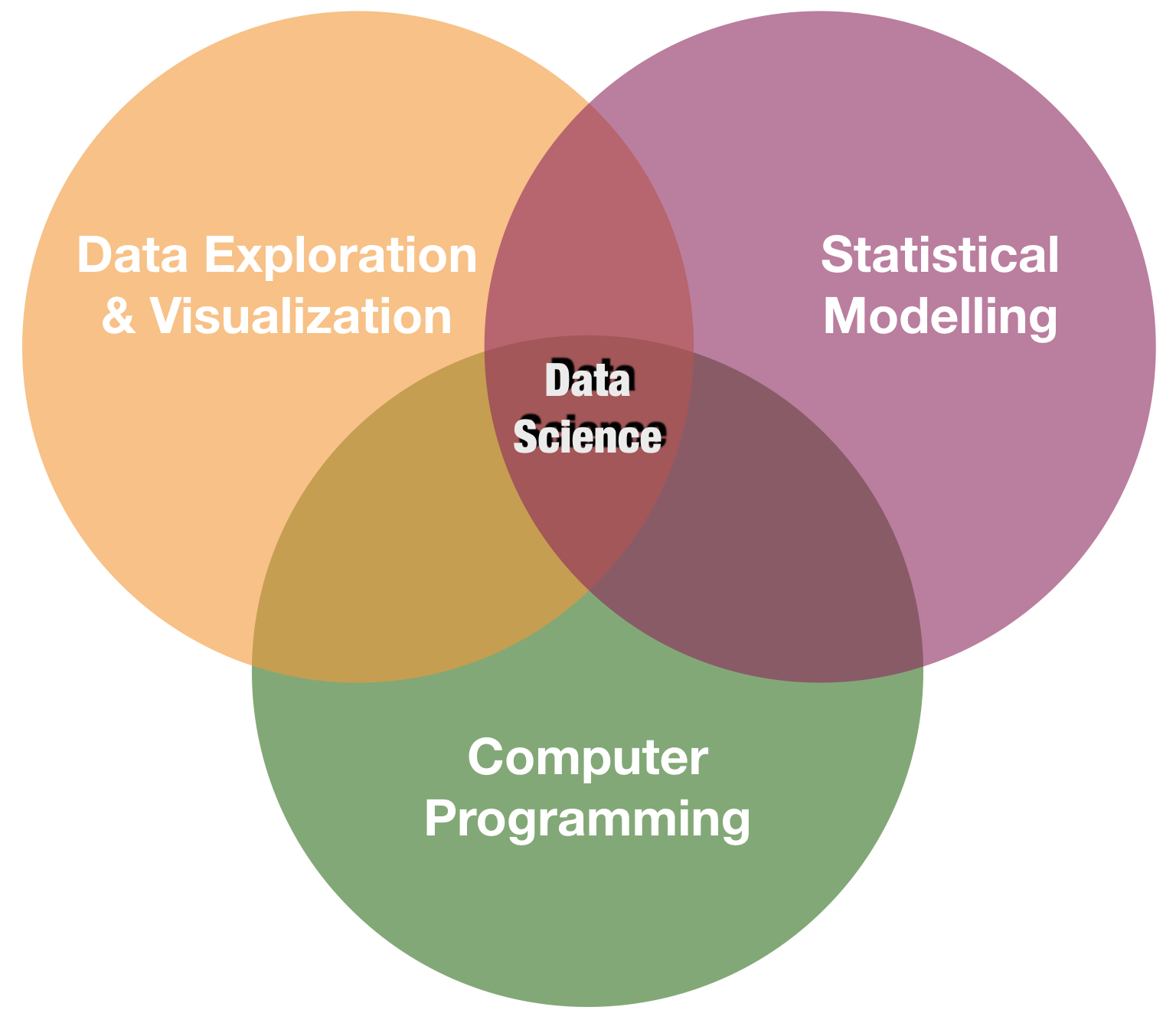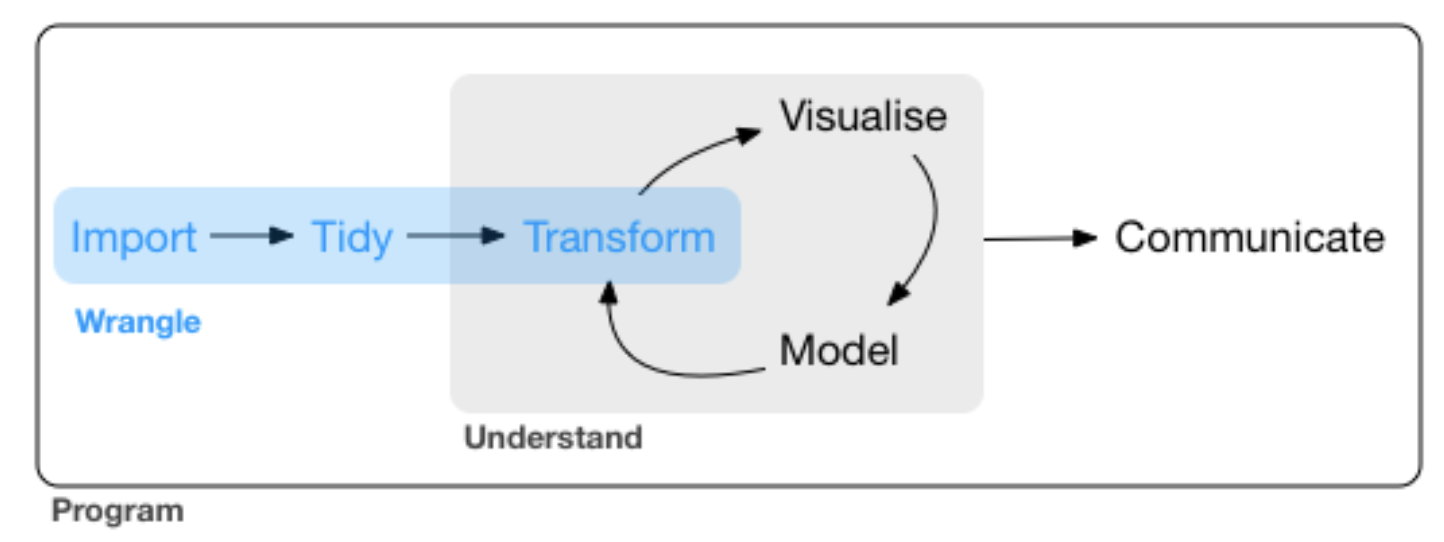source: R for Data Science by Wickam & Grolemund, 2017 (licensed under CC-BY-NC-ND 3.0 US)

p

A system for creating graphics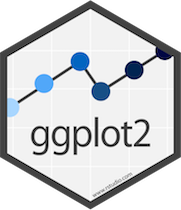• Based on "The Grammar of Graphics"
• The fundament for plotting in R nowadays
• Well documented
• Getting help: ggplot2 mailing list
• An increasing number of ggplot2 extensions developed by R users in the community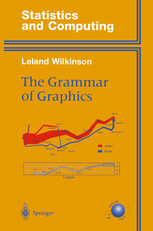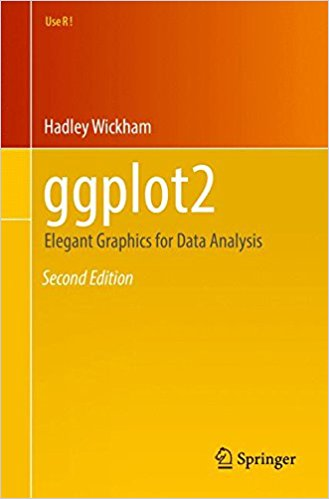Creating plots in a stepwise process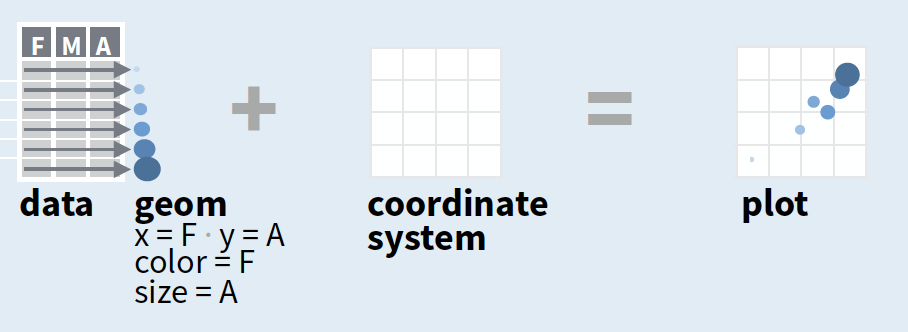• Start with ggplot(data, mapping = aes()) where you supply a dataset and (default) aesthetic mapping
• Add a layer by calling a geom_function
• Then add on (not required as defaults supplied)
• scales like xlim()
• faceting like facet_wrap()
• coordinate systems like coord_flip()
• themes like theme_bw()
• Save a plot to disk with ggsave()

source of image (topright): older version of Data Visualization with ggplot cheat sheet (licensed under CC-BY-SA)

Creating plots in a stepwise process• Start with ggplot(data, mapping = aes()) where you supply a dataset and (default) aesthetic mapping
• Add a layer by calling a geom_function
• Then add on (not required as defaults supplied)
• scales like xlim()
• faceting like facet_wrap()
• coordinate systems like coord_flip()
• themes like theme_bw()
• Save a plot to disk with ggsave()

aesthetic mapping:

to display values, variables in the data need to be mapped to visual properties of the geom (aesthetics) like size, color, and x and y locations. aes() mappings within ggplot() represent default settings for all layers (typically x and y), otherwise map variables within geom-functions.

Creating plots in a stepwise process• Start with ggplot(data, mapping = aes()) where you supply a dataset and (default) aesthetic mapping
• Add a layer by calling a geom_function
• Then add on (not required as defaults supplied)
• scales like xlim()
• faceting like facet_wrap()
• coordinate systems like coord_flip()
• themes like theme_bw()
• Save a plot to disk with ggsave()

geom_function:

combines a geometric object representing the observations with aesthetic mapping, a stat, and a position adjustment, e.g., geom_point() or geom_histogram()

Creating plots in a stepwise process• Start with ggplot(data, mapping = aes()) where you supply a dataset and (default) aesthetic mapping
• Add a layer by calling a geom_function
• Then add on (not required as defaults supplied)
• scales like xlim()
• faceting like facet_wrap()
• coordinate systems like coord_flip()
• themes like theme_bw()
• Save a plot to disk with ggsave()

scales:

control the details of how data values are translated to visual properties (override the default scales)

Creating plots in a stepwise process• Start with ggplot(data, mapping = aes()) where you supply a dataset and (default) aesthetic mapping
• Add a layer by calling a geom_function
• Then add on (not required as defaults supplied)
• scales like xlim()
• faceting like facet_wrap()
• coordinate systems like coord_flip()
• themes like theme_bw()
• Save a plot to disk with ggsave()

facetting:

smaller plots that display different subsets of the data; also useful for exploring conditional relationships.

Creating plots in a stepwise process• Start with ggplot(data, mapping = aes()) where you supply a dataset and (default) aesthetic mapping
• Add a layer by calling a geom_function
• Then add on (not required as defaults supplied)
• scales like xlim()
• faceting like facet_wrap()
• coordinate systems like coord_flip()
• themes like theme_bw()
• Save a plot to disk with ggsave()

coordinate system:

determines how the x and y aesthetics combine to position elements in the plot

Creating plots in a stepwise process• Start with ggplot(data, mapping = aes()) where you supply a dataset and (default) aesthetic mapping
• Add a layer by calling a geom_function
• Then add on (not required as defaults supplied)
• scales like xlim()
• faceting like facet_wrap()
• coordinate systems like coord_flip()
• themes like theme_bw()
• Save a plot to disk with ggsave()

themes:

control the display of all non-data elements of the plot. You can override all settings with a complete theme like theme_bw(), or choose to tweak individual settings

Creating plots in a stepwise process• Start with ggplot(data, mapping = aes()) where you supply a dataset and (default) aesthetic mapping
• Add a layer by calling a geom_function
• Then add on (not required as defaults supplied)
• scales like xlim()
• faceting like facet_wrap()
• coordinate systems like coord_flip()
• themes like theme_bw()
• Save a plot to disk with ggsave()

ggsave("plot.png", width = 5, height = 5):

Saves last plot as 5’ x 5’ file named "plot.png" in working directory. Matches file type to file extension.

A demonstration with the internal iris data

Step 1 - start a plot with ggplot()

ggplot(iris, aes(x = Sepal.Length,
y = Petal.Length))Step 2 - add layers: geom_point()

ggplot(iris, aes(x = Sepal.Length,
y = Petal.Length)) +
geom_point()Step 2 - add layers: geom_point()

ggplot(iris, aes(x = Sepal.Length,
y = Petal.Length)) +
geom_point(aes(col = Species))

aes(col = Species)

Show points in species-specific colours.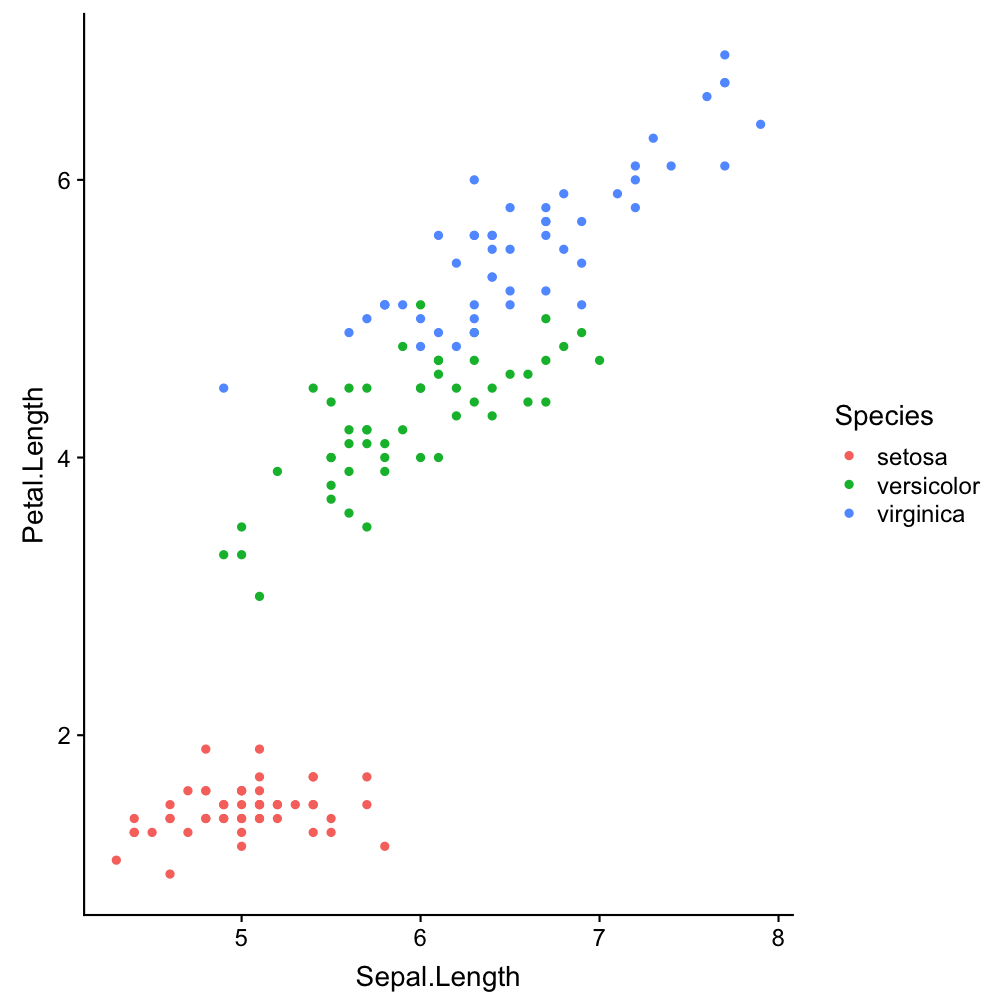Step 2 - add layers: geom_smooth()

ggplot(iris, aes(x = Sepal.Length,
y = Petal.Length)) +
geom_point(aes(col = Species)) +
geom_smooth()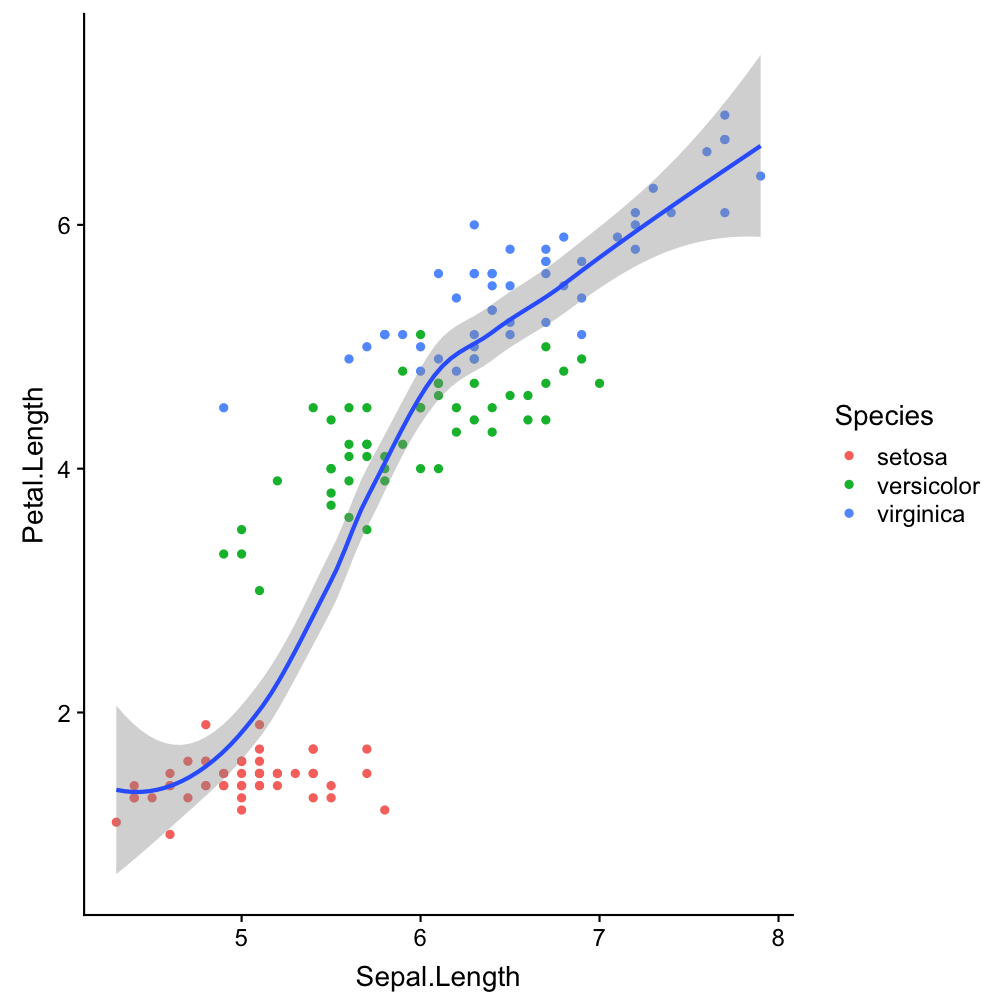Step 2 - add layers: geom_smooth()

ggplot(iris, aes(x = Sepal.Length,
y = Petal.Length)) +
geom_point(aes(col = Species)) +
geom_smooth(aes(col = Species),
method = "lm")

aes(col = Species), method = "lm"

Make smoother species-specific and linear.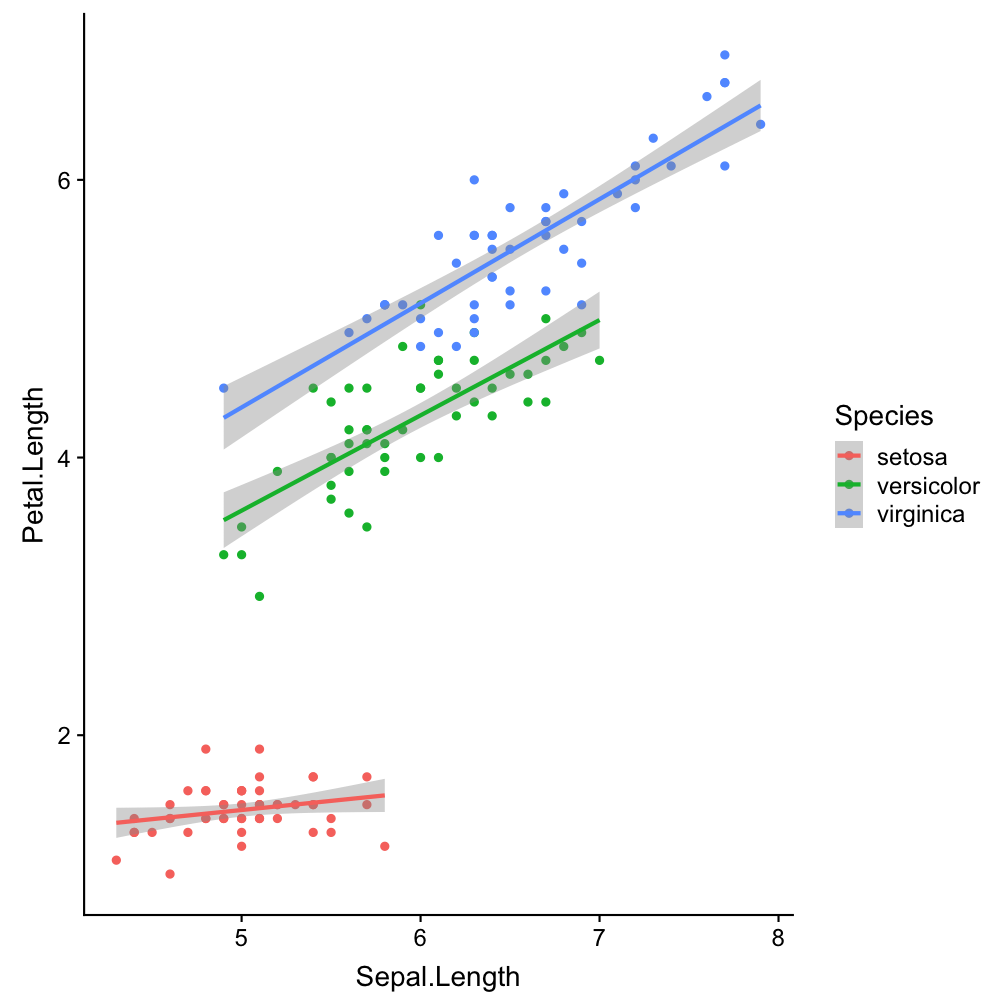Step 2 - add layers

ggplot(iris, aes(x = Sepal.Length,
y = Petal.Length, col = Species)) +
geom_point() +
geom_smooth(method = "lm")
Move species-specific colour aesthetics to ggplot() (so it becomes the default setting for all added layers).Step 3 - add scales: scale_colour_brewer()

ggplot(iris, aes(x = Sepal.Length,
y = Petal.Length, col = Species)) +
geom_point() +
geom_smooth(method = "lm") +
scale_colour_brewer()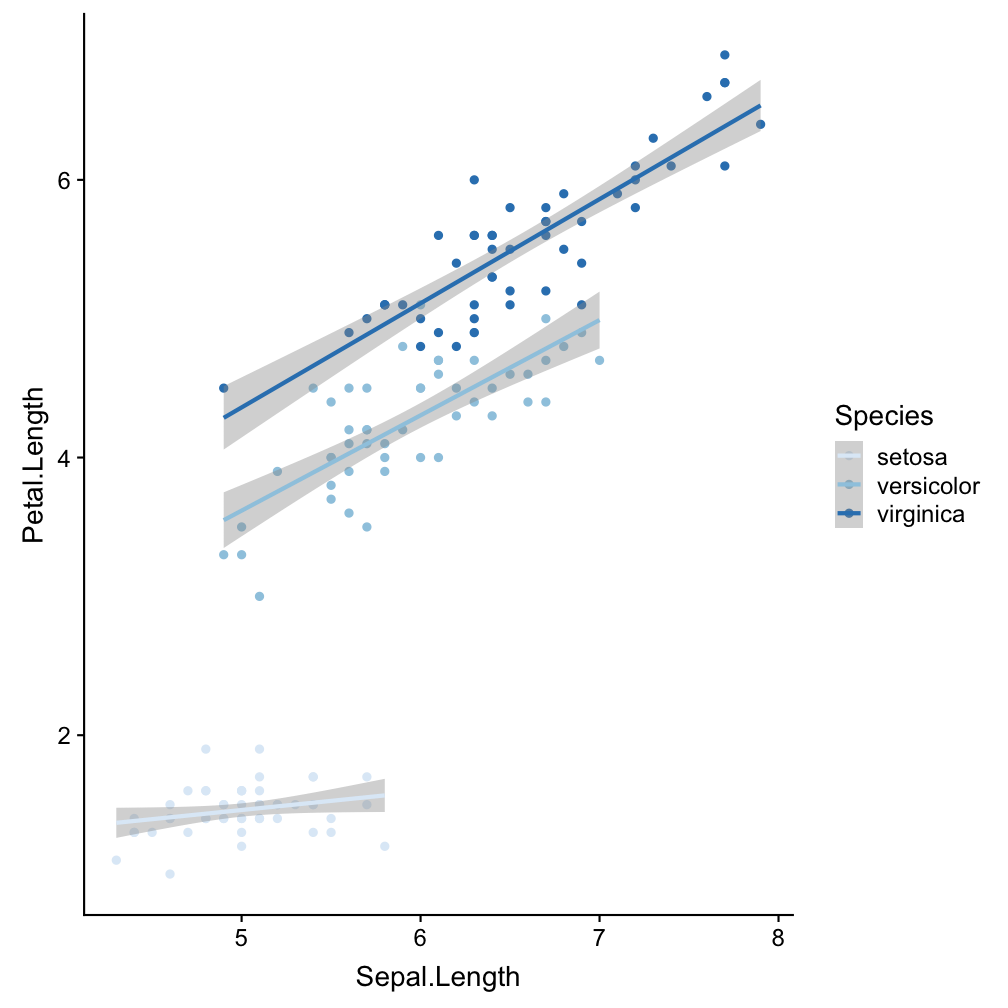Step 4 - add facets: facet_wrap()

ggplot(iris, aes(x = Sepal.Length,
y = Petal.Length, col = Species)) +
geom_point() +
geom_smooth(method = "lm") +
scale_colour_brewer() +
facet_wrap(~Species, nrow=3)

facet_wrap(~Species, nrow=3)

Divide the species-specific observations into different panels.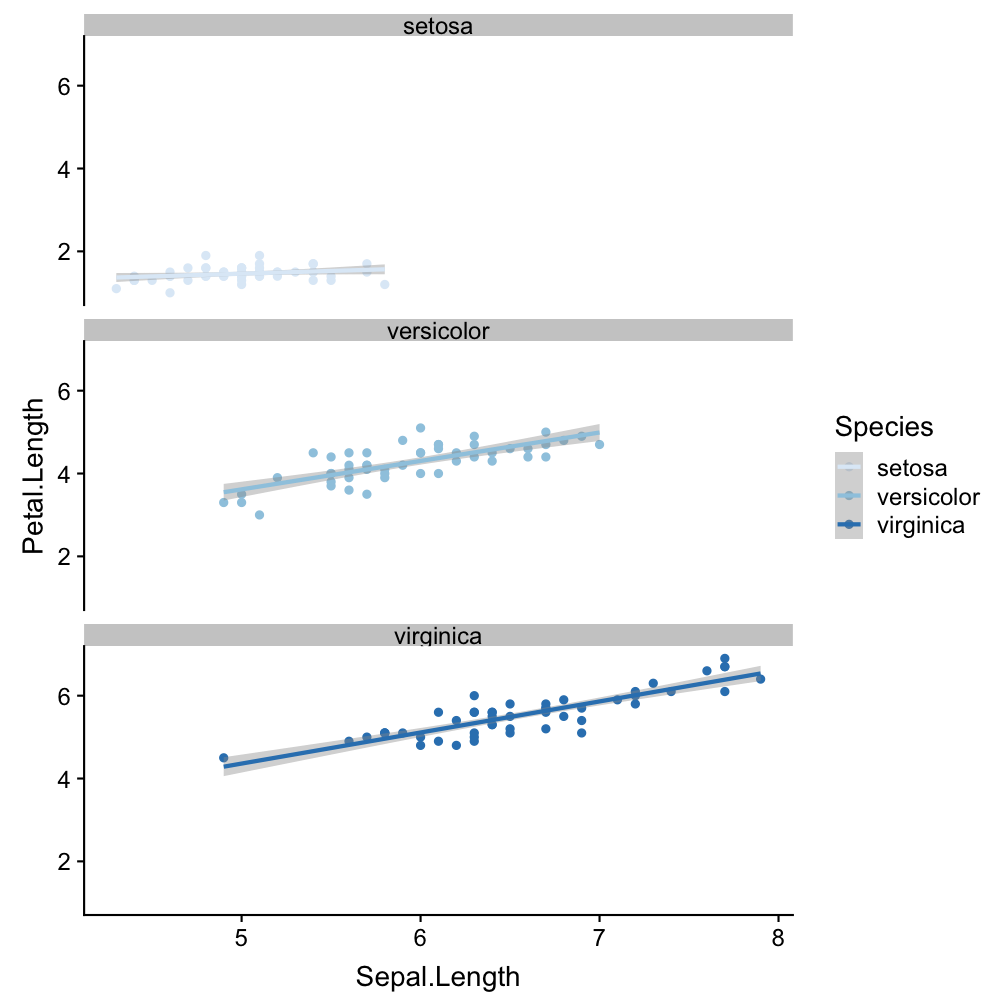Step 5 - modify coordinate system: coord_polar()

ggplot(iris, aes(x = Sepal.Length,
y = Petal.Length, col = Species)) +
geom_point() +
geom_smooth(method = "lm") +
scale_colour_brewer() +
facet_wrap(~Species, nrow=3) +
coord_polar()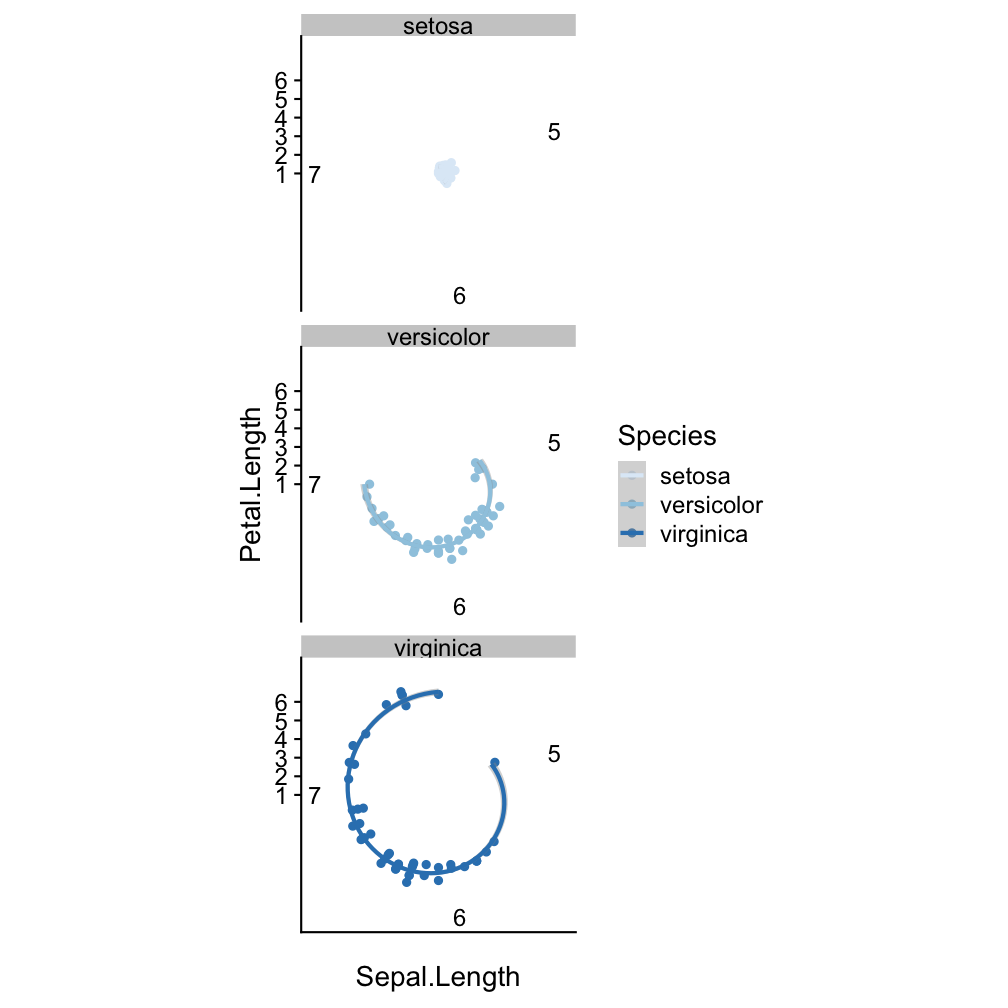Step 6 - change the look of non-data elements: theme_dark()

ggplot(iris, aes(x = Sepal.Length,
y = Petal.Length, col = Species)) +
geom_point() +
geom_smooth(method = "lm") +
scale_colour_brewer() +
facet_wrap(~Species, nrow=3) +
coord_polar() +
theme_dark()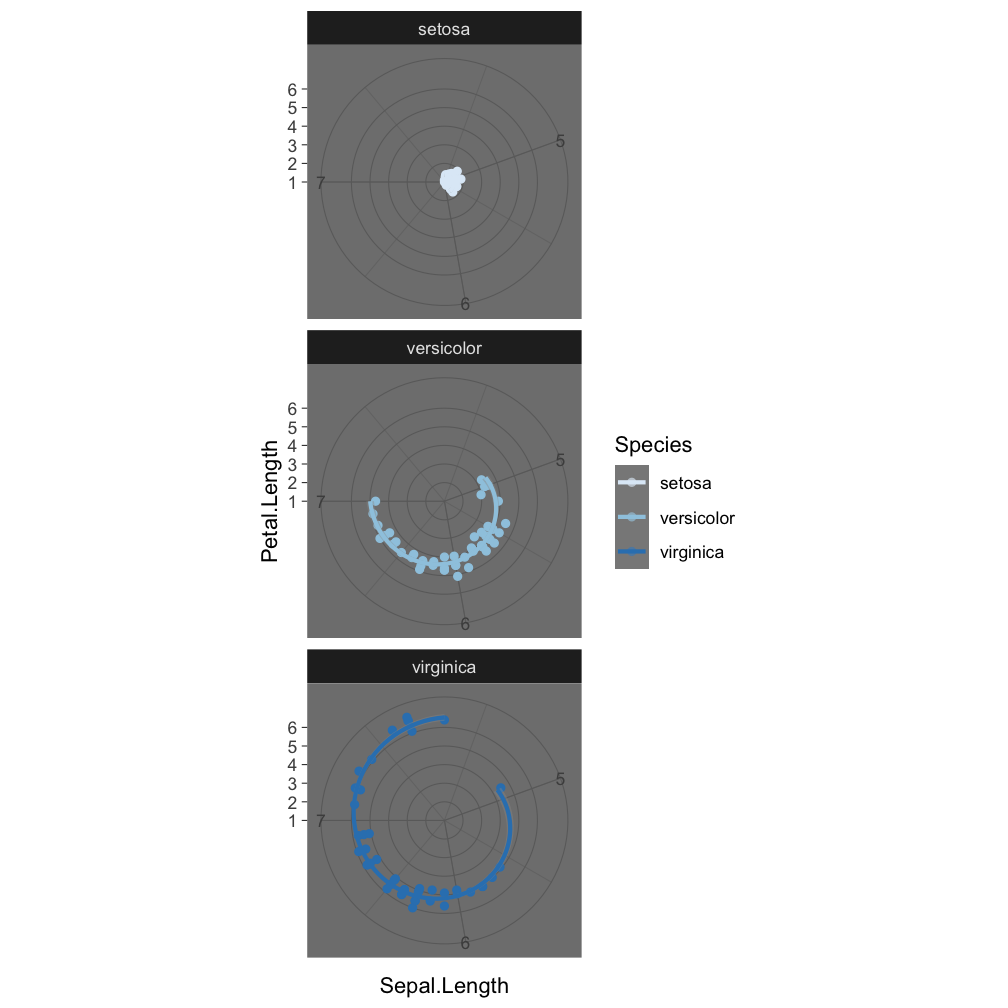Step 7 - save the plot: ggsave()

ggplot(iris, aes(x = Sepal.Length,
y = Petal.Length, col = Species)) +
geom_point() +
geom_smooth(method = "lm") +
scale_colour_brewer() +
facet_wrap(~Species, nrow=3) +
coord_polar()

ggsave("Iris_length_relationships.pdf", width = 4, height = 4)

The last plot displayed is saved (as default).

ggplot - geom_functions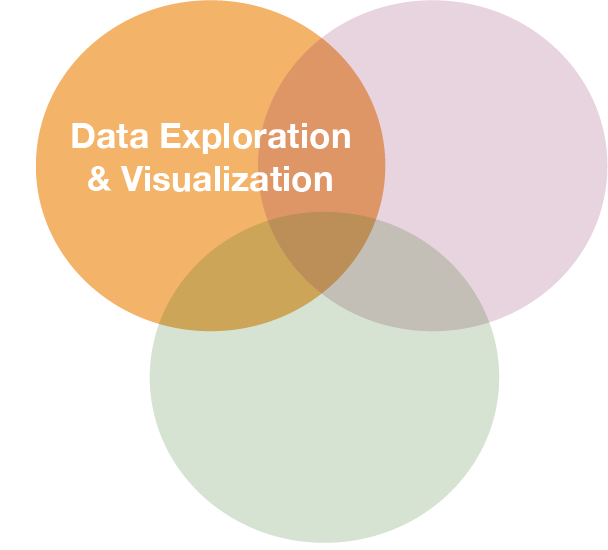When to use which function?

That depends on

• what you want to display (your question)
• and the type of data

Common plots are ...

Visualize frequency distributions

BARPLOTS -
are used for categorical or discrete variables. Bars do not touch each other; there are no ‘in-between’ values.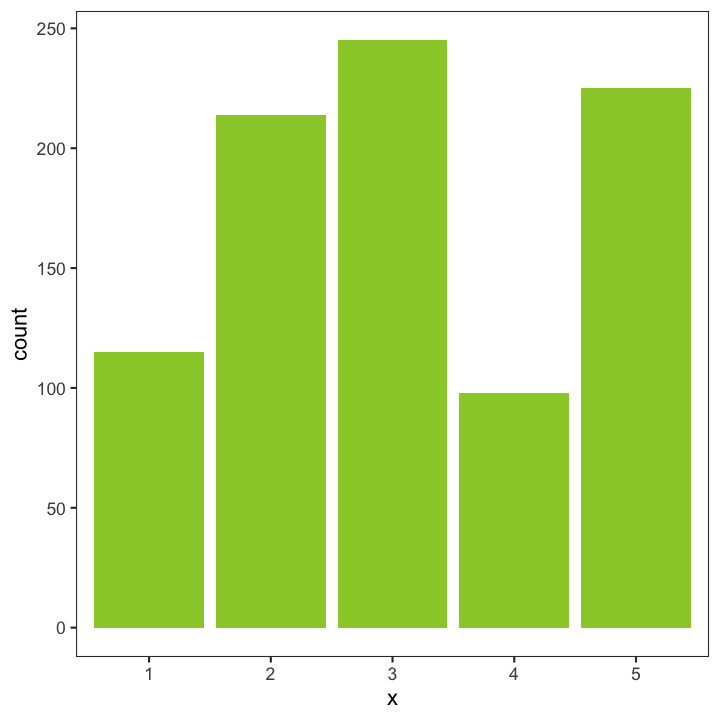HISTOGRAMS and DENSITY PLOTS (can be combined) are used for continuous variables and are often used to check whether variables are normally distributed. Bars touch each other in histograms.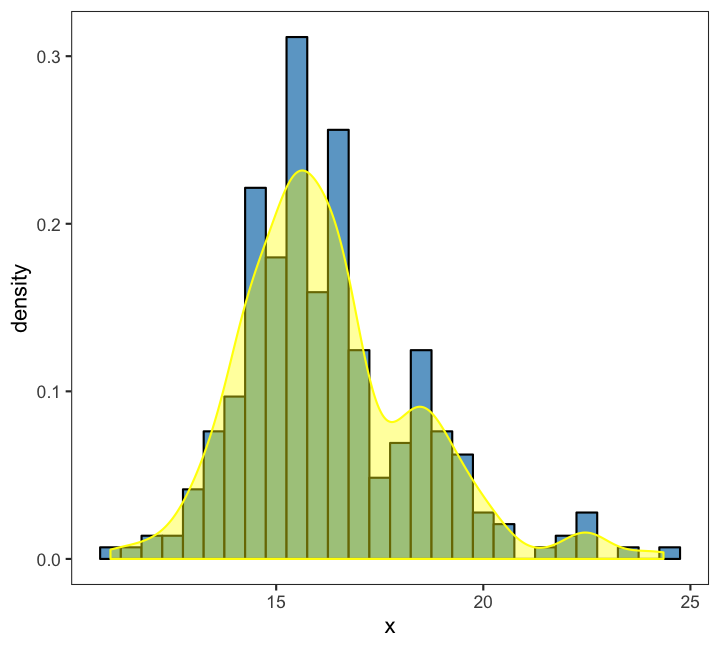Compare groups

BOXPLOTS - are used to compare two or more groups in terms of their distributional center and spread. They transport a lot of information and should be computed in every data exploration! You can check,

• differences in average y values between groups and whether these might be significant,
• if group variances differ,
• whether individual groups are normally distributed
• identify outlier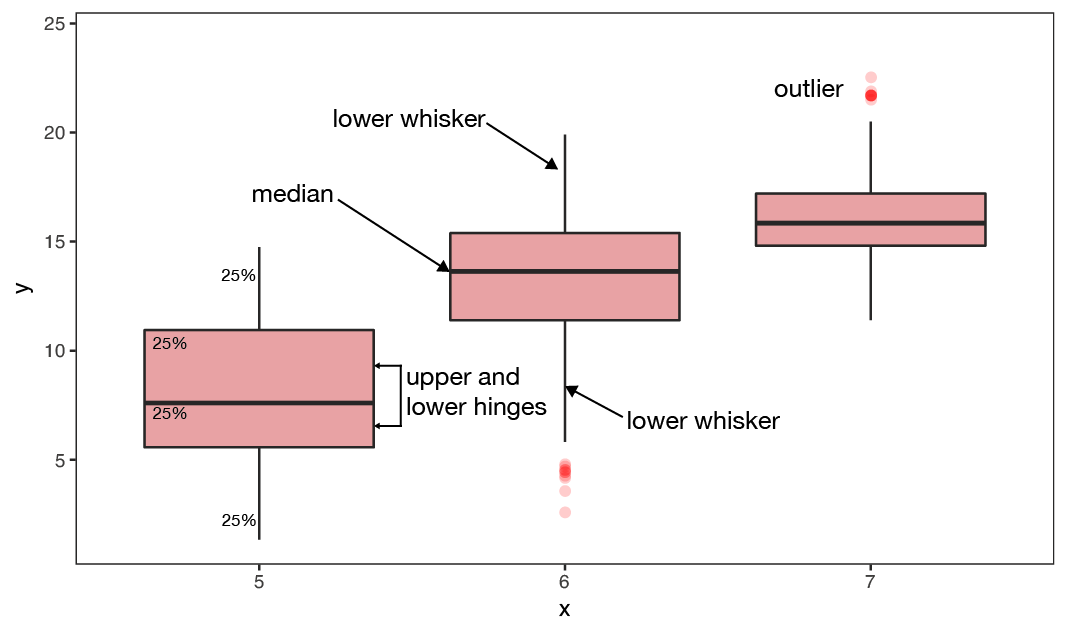Show relationship between 2 variables

SCATTERPLOTS -
are the most basic plots for continuous variables. They are also the least interpreting plots as they show every observation in the 2-dimensional space.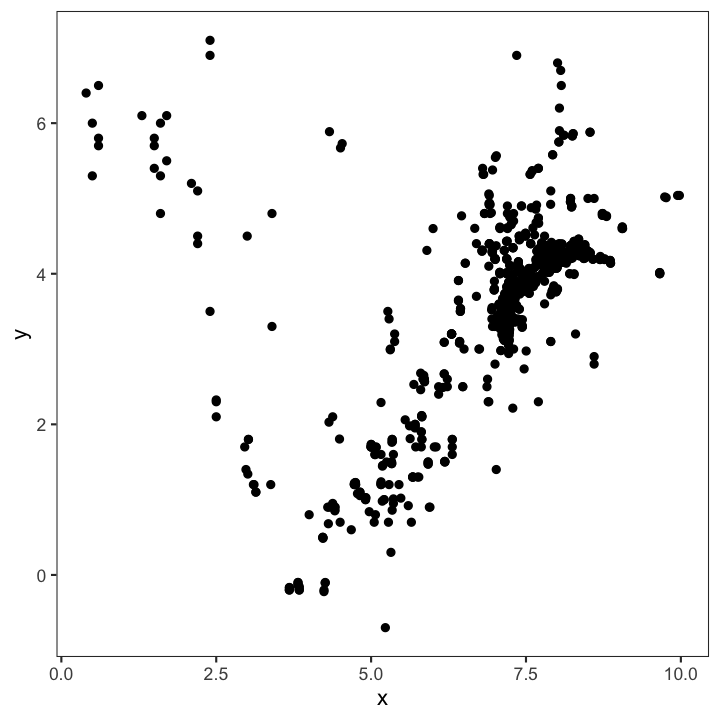Another useful feature is that they can be combined with other plotting elements: defining aesthetics for a 3rd variable (e.g. colours of points) or adding regression or smoothing lines to help visualise the relationship: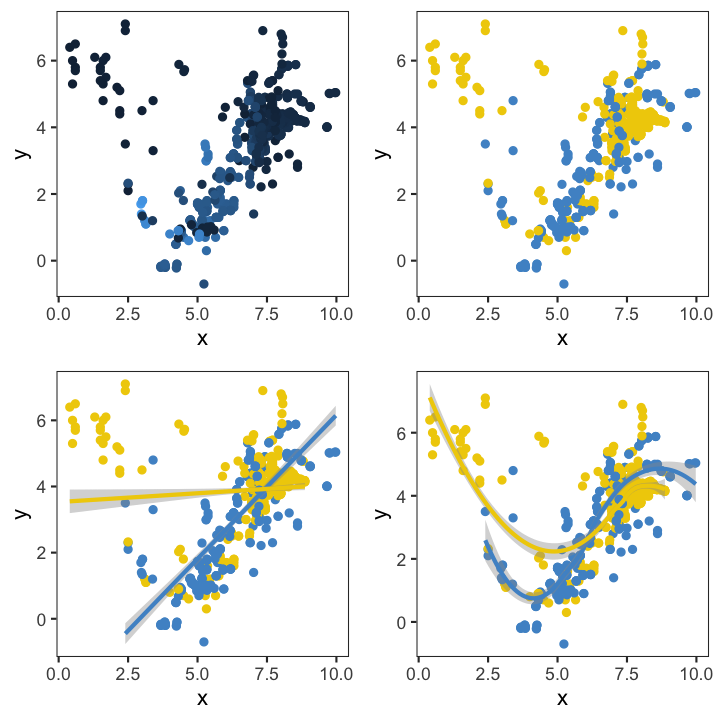An overview of core geom_functions depending on the type of data:

Elements taken from older version of ggplot cheat sheet

Some examples with the ICES hydro dataA barplot to see the frequency of monthly samplings

hydro_sub <- hydro %>%
select(fmonth, station, date_time) %>%
# (fmonth = month as factor)
distinct()

ggplot(hydro_sub,aes(x=fmonth)) +
geom_bar()

Note

For barplots (or boxplots) x must be categorical, hence, we use here month as a factor!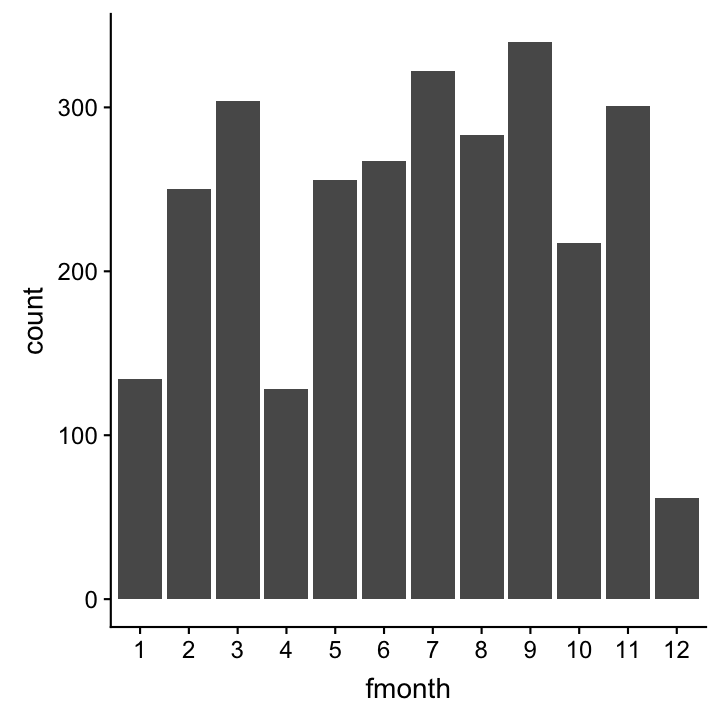A scatterplot to see all stations, coloured by month

Note:

To get a discrete scale we use again fmonth.
hydro_sub <- hydro %>%
select(fmonth,station,lat,long) %>%
distinct()

ggplot(hydro_sub, aes(x = long,
y = lat, col = fmonth)) +
geom_point()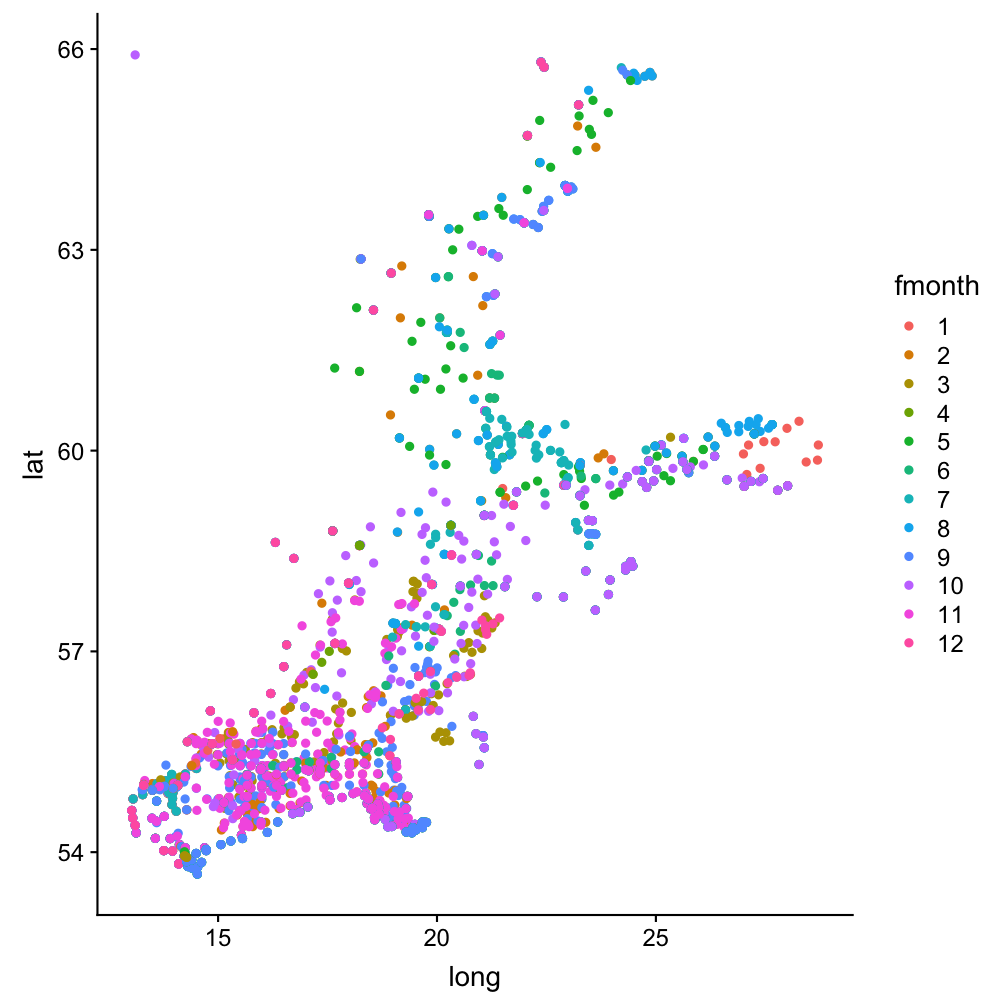A histogram to see the distribution of (all) temperature values

Note:

You can use the pipe operator also when plotting and saving ggplot as an object!
p <- hydro %>% ggplot(aes(x = temp)) +
geom_histogram()
p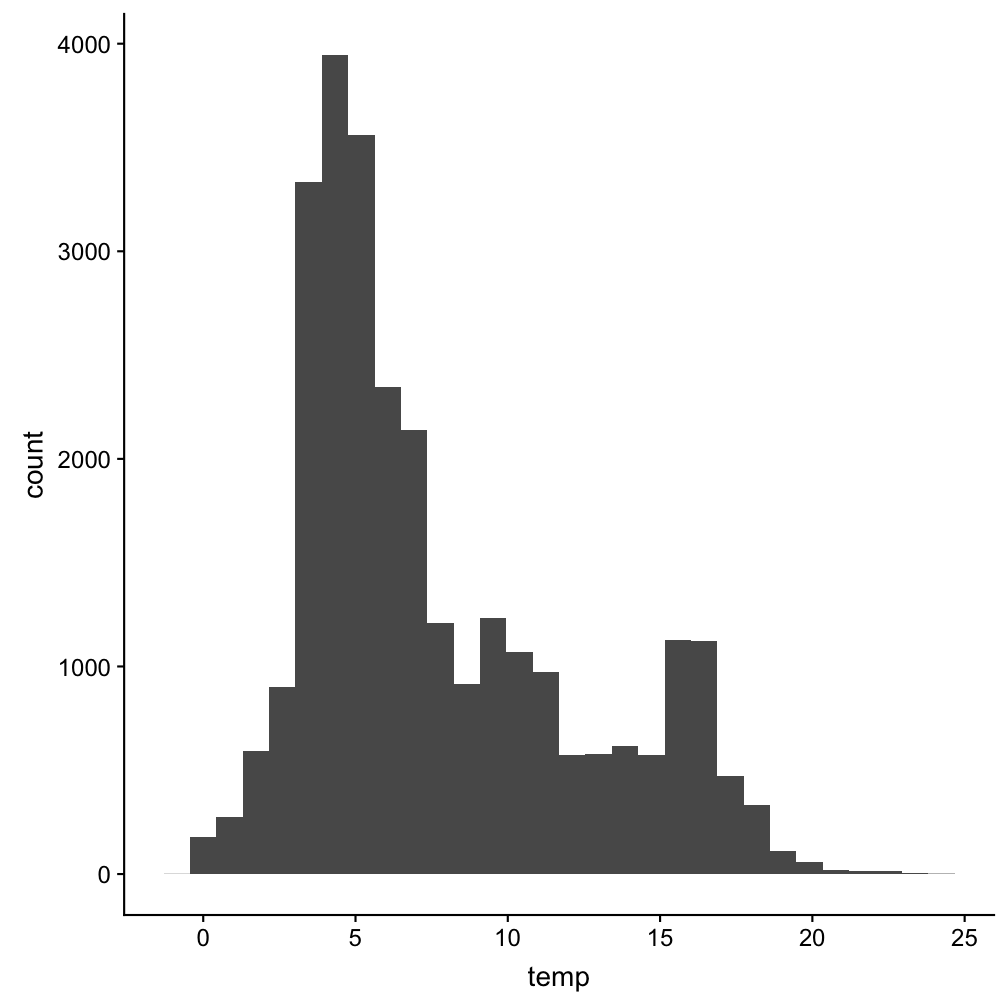A boxplot to compare the surface temp between months

hydro %>% filter(pres < 5) %>%
group_by(fmonth, station, date_time, cruise) %>%
summarise(mean_sst = mean(temp)) %>% ungroup() %>%
ggplot(aes(x = fmonth, y = mean_sst)) +
geom_boxplot(outlier.colour = "red", outlier.alpha = 0.2)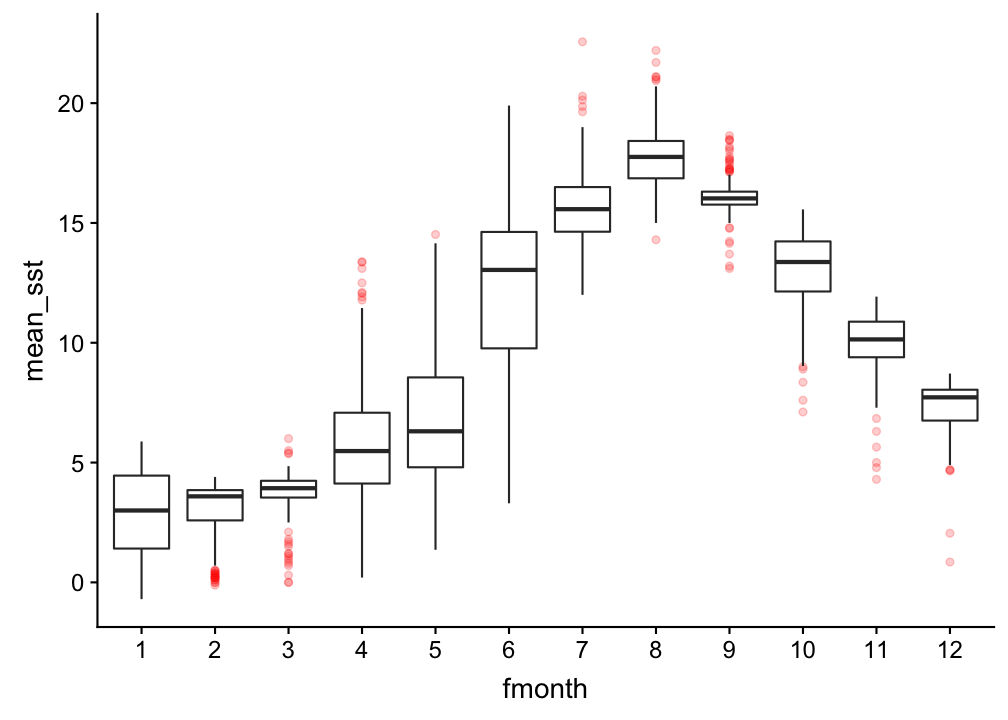Correlation plot between temperature and salinity

ggplot(hydro, aes(x = psal, y = temp, col = day)) +
geom_point()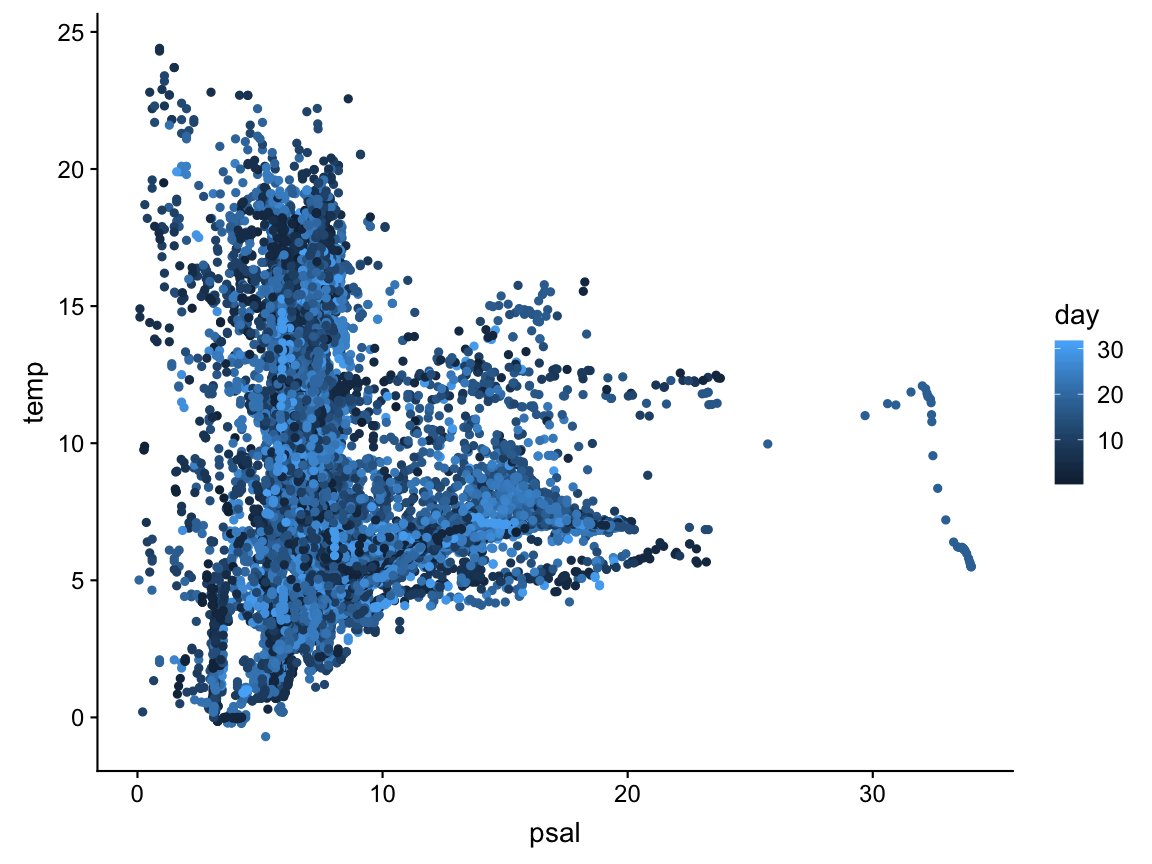.. now separated by month

ggplot(hydro,
aes(x = psal,
y = temp,
col = day)) +
geom_point() +
facet_wrap(
~fmonth,
nrow = 3)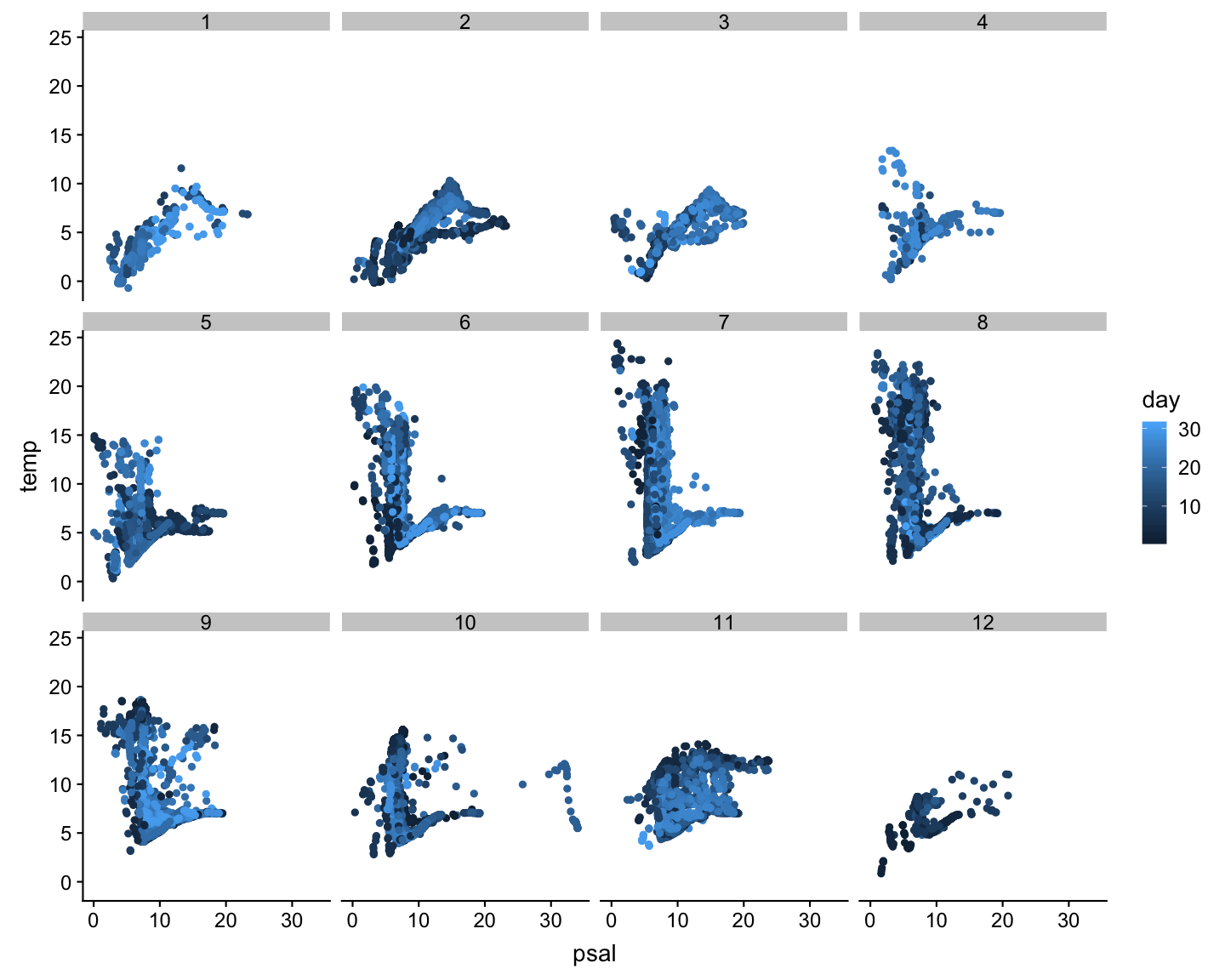Depth profile at one station in July

hydro_sub <- hydro %>%
filter(station=="0403",fmonth==7,day==11)
p_temp <- ggplot(hydro_sub, aes(y=pres)) +
geom_point(aes(x = temp), col="red") +
ylim(70, 0)
p_sal <- ggplot(hydro_sub, aes(y=pres)) +
geom_point(aes(x = psal)) +
ylim(70, 0)
p_oxy <- ggplot(hydro_sub, aes(y=pres)) +
geom_point(aes(x = doxy), col="blue") +
ylim(70, 0)
gridExtra::grid.arrange(grobs = list(
p_temp, p_sal, p_oxy), nrow=1)

Note

The colour specification is outside the aesthetic mapping: because we want the same colour for all observations.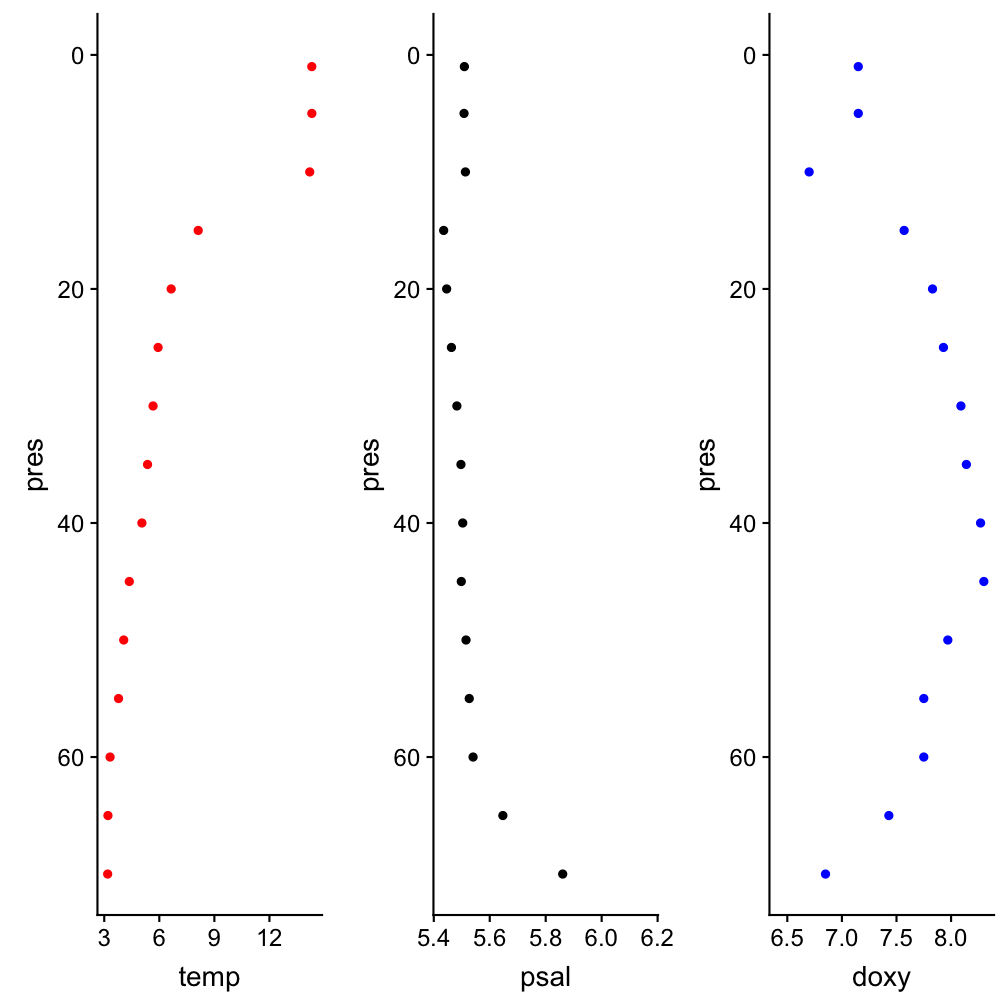Some extension examples: ggridges

library(ggridges)
hydro %>% filter(pres < 10) %>%
group_by(station, fmonth, day) %>%
summarise(
sst = mean(temp, na.rm = TRUE)) %>%
group_by(station, fmonth) %>%
summarise(
sst = mean(sst, na.rm = TRUE)) %>%
ungroup() %>%
ggplot(aes(x = sst, y = fmonth,
fill = fmonth)) +
geom_density_ridges(scale = 12,
rel_min_height = 0.005) +
scale_fill_cyclical(
values = c("blue", "green")) +
theme_ridges()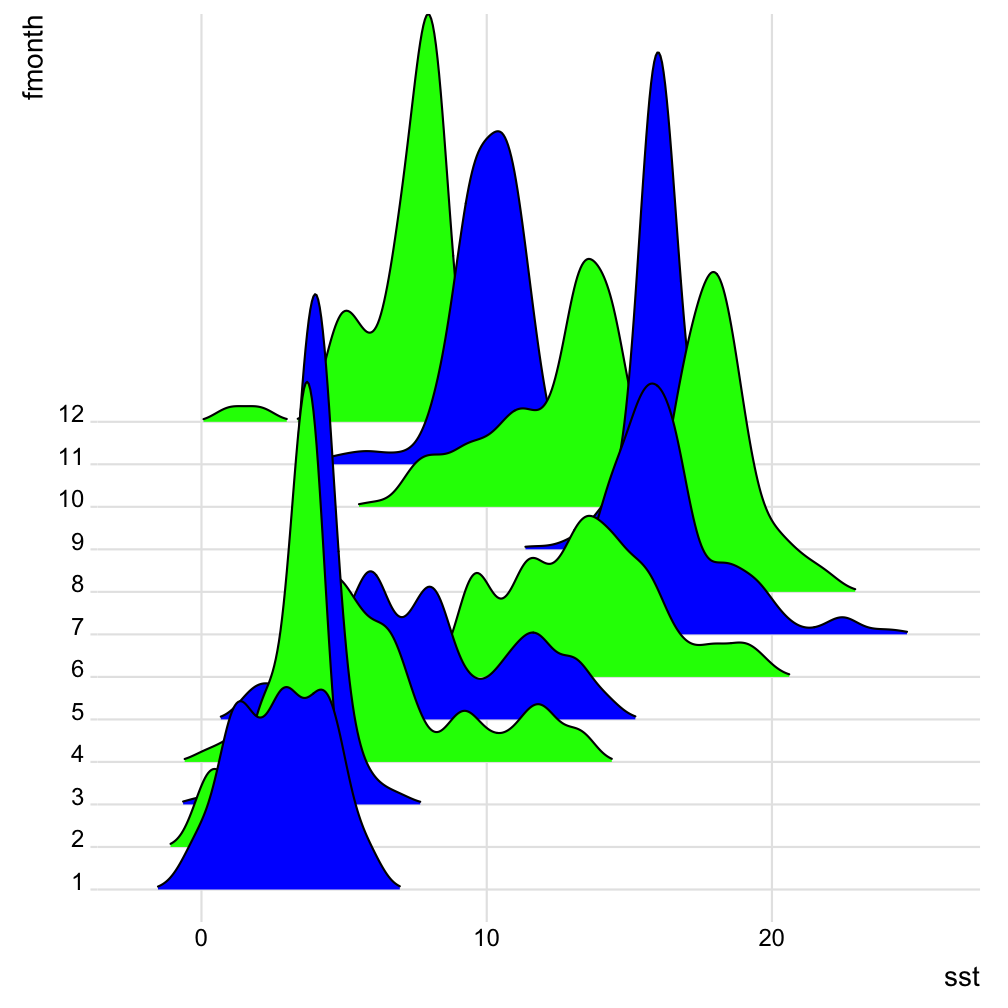Some extension examples: ggmap

library(ggmap)
b <- matrix(c(
min(hydro$long), max(hydro$long),
min(hydro$lat), max(hydro$lat) ), byrow=T,nrow=2)
colnames(b) <- c("min","max")
rownames(b) <- c("x","y")
map_bs <- ggmap(get_map(location = b,
zoom = 5))
map_bs + geom_point(data=hydro,
aes(long,lat), size=0.5,
color="red")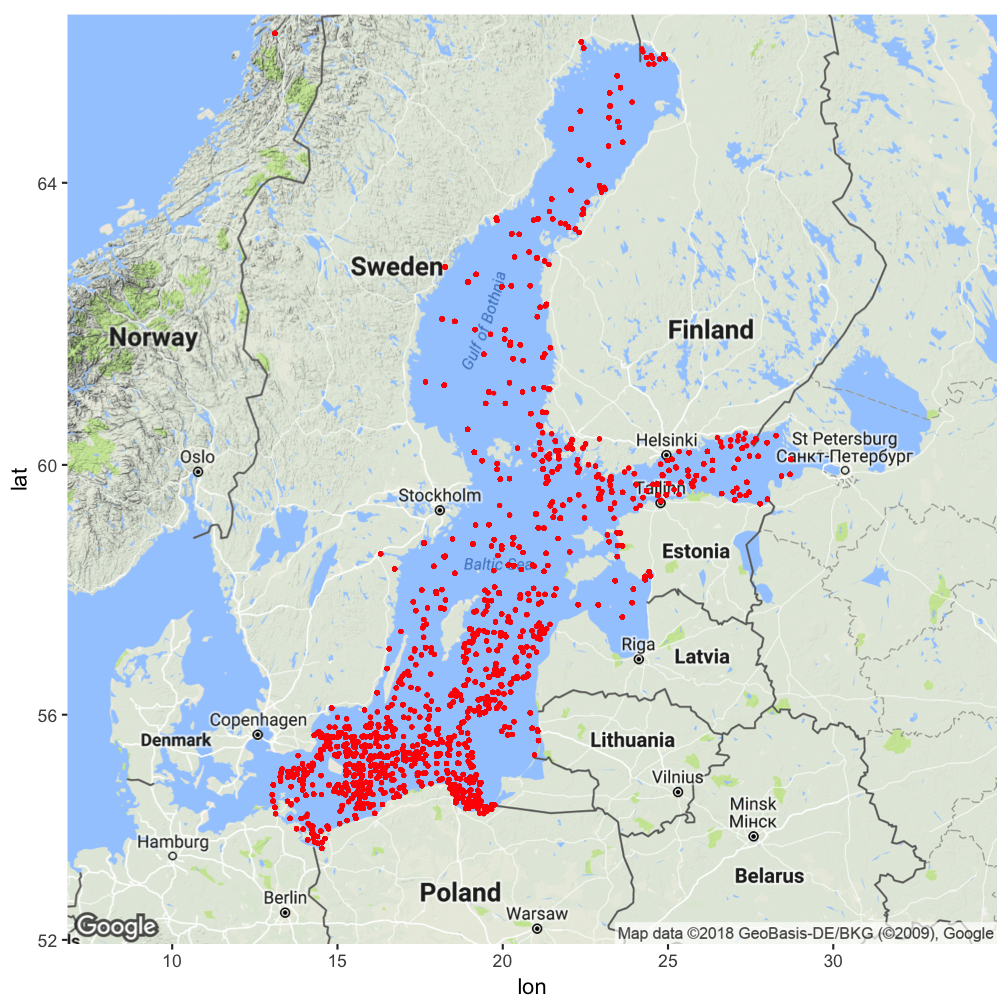Some extension examples: ggmap

baltic <- c(left = min(hydro$long), bottom = min(hydro$lat),
right = max(hydro$long), top = max(hydro$lat))
map_stamen <- get_stamenmap(baltic,
zoom = 5, maptype = "toner-lite")
ggmap(map_stamen) +
geom_point(data=hydro,
aes(long,lat), size=0.5,
color="red") + theme_linedraw()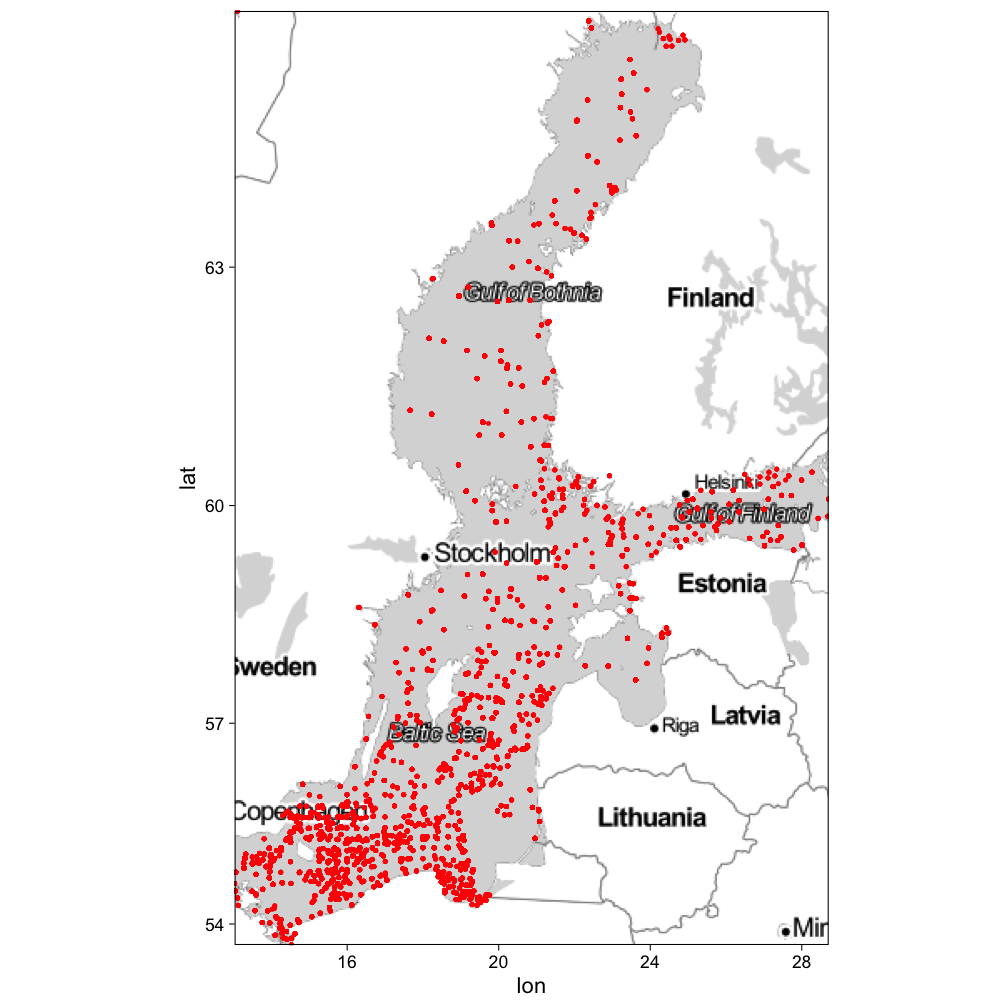Quiz 1: Syntax

Complete the following code snippet (fill in the ... gaps) to create the plot you need for answering the question below.

library(ggplot2)

p <- ...(... = mtcars, ...(wt, mpg, label = rownames(mtcars)))
... + geom_point(...(size = gear)) ...
geom_text(...(colour = factor(cyl)), hjust = 0, nudge_... = 0.05)
1. Find the car model in the 'mtcars' dataset with the lowest mpg (=Miles per(US) gallon) value in its 4 cyl(inder) class that also has the lowest number of (forward) gears. Which position in the alphabet has the first letter of this model?

You have to fill in 4 times a function name, 2 times a (partial) argument, 1 symbol (which is in fact also a function), and 1 object name.

1. 20

Quiz 2: Get to know geom_functions

Which of these function is NOT a geom_function?

1. geom_hex
2. geom_linerange
3. geom_label
4. geom_ribbon
5. geom_trend
6. geom_sf
7. geom_crossbar

The webside http://ggplot2.tidyverse.org/reference/ provides an overview of all geom_functions

Small exercises with the ICES hydro dataset

1. What happens if you make a scatterplot of station (x) vs temp (y)? Why is the plot not useful? What would be a better plot?
2. What happens if you make a boxplot of cruise (x) vs psal (y)? Why is this plot less suitable? What could be an alternative?

How do you feel now.....?

Totally confused?Try to reproduce some of the plots in this presentation and the quiz and read chapter 3 on data visualization in 'R for Data Science' .

Totally bored?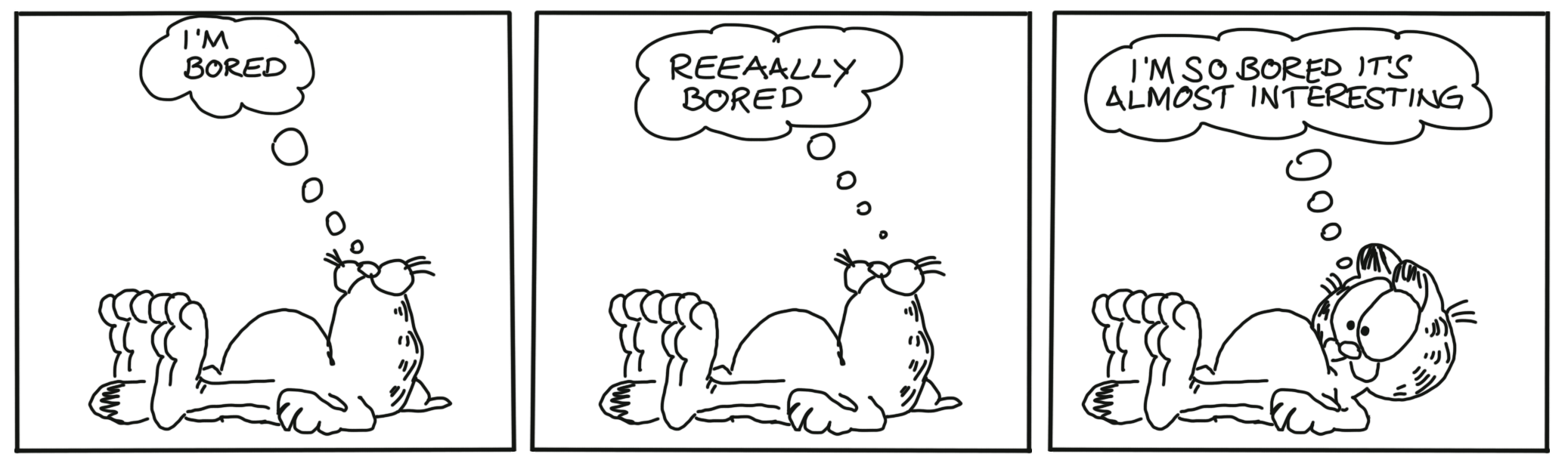Then figure out how to get a CTD profile in ONE panel!

Totally content?

Then go grab a coffee, lean back and enjoy the rest of the day...!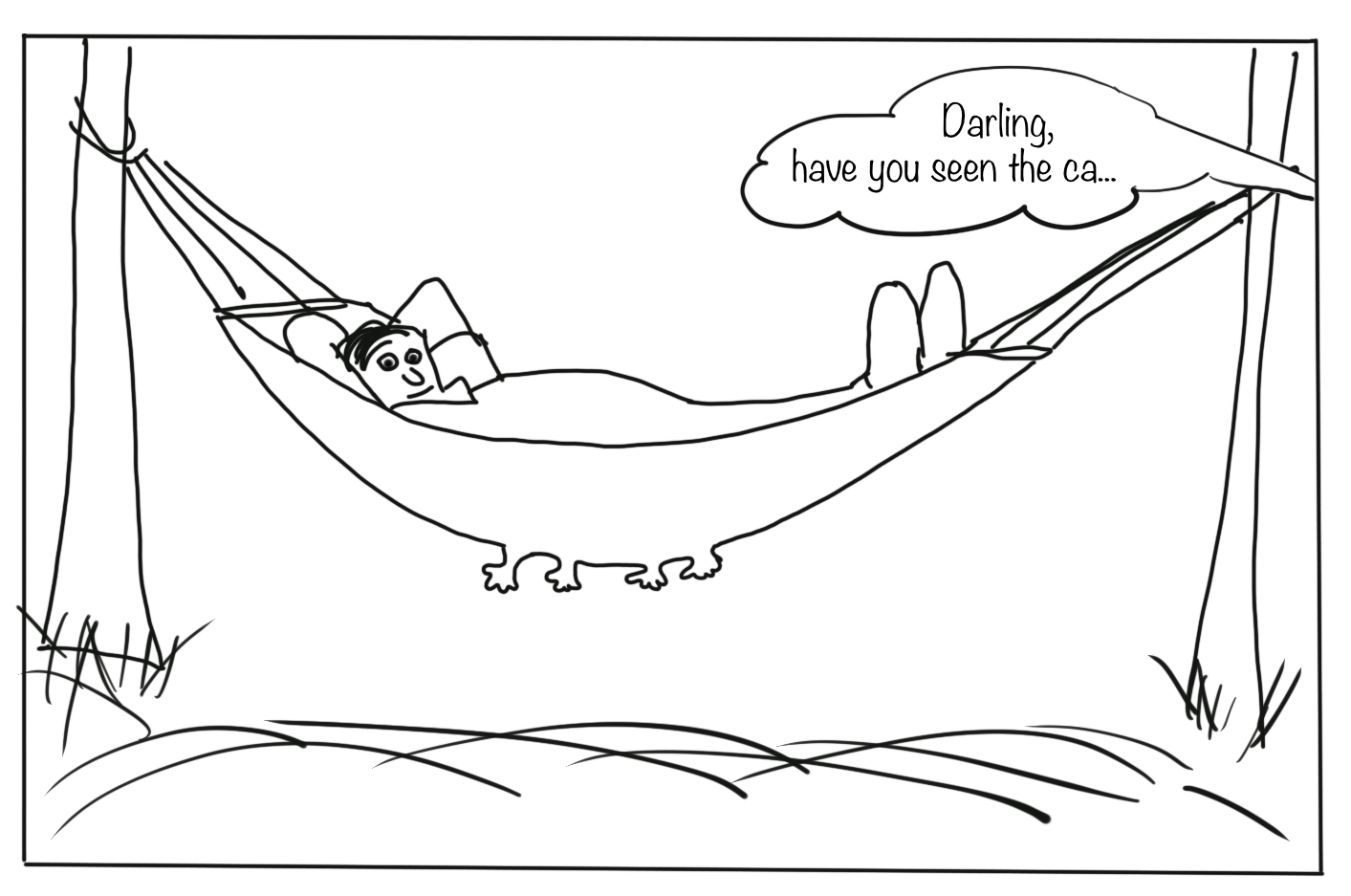Thank You